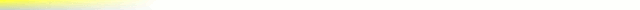# [mkgmap-dev] Commit r3680: reduceImgSize_v2.patch :

From svn commit svn at mkgmap.org.uk on Wed Jul 20 06:52:33 BST 2016

```Version mkgmap-r3680 was committed by gerd on Wed, 20 Jul 2016

reduceImgSize_v2.patch :
- Implement the "trick" to store delta values which require more bits than the "base" value says
- Try different base values to find out if the trick reduces the length of the bit stream
The patch reduces the image size by up to 13%, the additional iterations may require a few percent
more run time.

A real world example that shows how the "trick" works:
https://www.openstreetmap.org/way/245112355

This highway has 6 points, it starts in the north, the x-delta values (in resolution 24) are
4, -19, -5, 0,  3
The largest absolute value is 19 which requires 5 bits: 10011.
Since both positive and negative values exist, we have to encode the sign for each single delta value, so we have to use 6 bits (the leftmost gives the sign)
So, the first iteration gives:
6 bit words : 4 = 000100  , -19 = 110011, -5 = 111011, 0 = 000000, 3 = 000011   --> 30 bits
Now, the optimization process starts trying to use the "trick" with 5 bits. WIth the trick,
the value 19 doesn't fit into 5 bits, so the trick is used (it stands for the binary value 1111) and the result for -19 is 10000 11100  (15 + 4  made negative)
All other values fit into 5 bits, so the 2nd iteration gives:
5 bit words: 4 = 00100  , -19 = 10000 11100, -5 = 11011, 0 = 00000, 3 = 00011   --> 30 bits
The next step is to try with only 4 bits. Again, only the value -19 needs the trick, this time we get - 19 = 1000 1000 1011 (7 +7 + 5 made negative)

4 bit words : 4 = 0100  , -19 = 1000 1000 1011, -5 = 1011, 0 = 0000, 3 = 0011   --> 24 bits

The next step is to try with only 3 bits. This time, more values need the trick (4 , -5 and -19). For -19 we get 100 100 100 100 100 100 111 (3 + 3 + 3 + 3 + 3+ 3 + 1 made negative)
The result:
3 bit words : 4 = 100 001 , -19 = 100 100 100 100 100 100 111, -5 = 100 110, 0 = 000, 3 = 011   --> 39 bits

So, we see that the trick is used too often now and the resulting bit stream is much longer than the one before.

So, using the trick with 4 bit words saves 6 bits for the x values.

http://www.mkgmap.org.uk/websvn/revision.php?repname=mkgmap&rev=3680
```